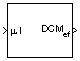Documentation

# Direction Cosine Matrix ECEF to NED

Convert geodetic latitude and longitude to direction cosine matrix

## Library

Utilities/Axes Transformations## Description

The Direction Cosine Matrix ECEF to NED block converts geodetic latitude and longitude into a 3-by-3 direction cosine matrix (DCM). The DCM matrix performs the coordinate transformation of a vector in Earth-centered Earth-fixed (ECEF) axes (ox0, oy0, oz0) into a vector in north-east-down (NED) axes (ox2, oy2, oz2). The order of the axis rotations required to bring this about is:

1. A rotation about oz0 through the longitude (ι) to axes (ox1, oy1, oz1)

2. A rotation about oy1 through the geodetic latitude (μ) to axes (ox2, oy2, oz2)

`$\begin{array}{l}\left[\begin{array}{c}o{x}_{2}\\ o{y}_{2}\\ o{z}_{2}\end{array}\right]=DC{M}_{ef}\left[\begin{array}{c}o{x}_{0}\\ o{y}_{0}\\ o{z}_{0}\end{array}\right]\\ \\ \left[\begin{array}{c}o{x}_{2}\\ o{y}_{2}\\ o{z}_{2}\end{array}\right]=\left[\begin{array}{ccc}-\mathrm{sin}\mu & 0& \mathrm{cos}\mu \\ 0& 1& 0\\ -\mathrm{cos}\mu & 0& -\mathrm{sin}\mu \end{array}\right]\left[\begin{array}{ccc}\mathrm{cos}\iota & \mathrm{sin}\iota & 0\\ -\mathrm{sin}\iota & \mathrm{cos}\iota & 0\\ 0& 0& 1\end{array}\right]\left[\begin{array}{c}o{x}_{0}\\ o{y}_{0}\\ o{z}_{0}\end{array}\right]\end{array}$`

Combining the two axis transformation matrices defines the following DCM.

`$DC{M}_{ef}=\left[\begin{array}{ccc}-\mathrm{sin}\mu \mathrm{cos}\iota & -\mathrm{sin}\mu \mathrm{sin}\iota & \mathrm{cos}\mu \\ -\mathrm{sin}\iota & \mathrm{cos}\iota & 0\\ -\mathrm{cos}\mu \mathrm{cos}\iota & -\mathrm{cos}\mu \mathrm{sin}\iota & -\mathrm{sin}\mu \end{array}\right]$`

## Inputs and Outputs

InputDimension TypeDescription

First

2-by-1 vectorContains the geodetic latitude and longitude, in degrees. Latitude and longitude values can be any value. However, latitude values of +90 and -90 may return unexpected values because of singularity at the poles.
OutputDimension TypeDescription

First

3-by-3 direction cosine matrixTransforms ECEF vectors to NED vectors.

## Assumptions

The implementation of the ECEF coordinate system assumes that the origin is at the center of the planet, the x-axis intersects the Greenwich meridian and the equator, the z-axis is the mean spin axis of the planet, positive to the north, and the y-axis completes the right-hand system.

## References

Stevens, B. L., and F. L. Lewis, Aircraft Control and Simulation, John Wiley & Sons, New York, 1992.

Zipfel, P. H., Modeling and Simulation of Aerospace Vehicle Dynamics, AIAA Education Series, Reston, Virginia, 2000.

“Atmospheric and Space Flight Vehicle Coordinate Systems,” ANSI/AIAA R-004-1992.

#### Introduced before R2006a

##### SupportGet trial now